Courses

# Classification Quiz I, Non Verbal Reasoning

## 25 Questions MCQ Test Non Verbal Reasoning | Classification Quiz I, Non Verbal Reasoning

Description
This mock test of Classification Quiz I, Non Verbal Reasoning for LR helps you for every LR entrance exam. This contains 25 Multiple Choice Questions for LR Classification Quiz I, Non Verbal Reasoning (mcq) to study with solutions a complete question bank. The solved questions answers in this Classification Quiz I, Non Verbal Reasoning quiz give you a good mix of easy questions and tough questions. LR students definitely take this Classification Quiz I, Non Verbal Reasoning exercise for a better result in the exam. You can find other Classification Quiz I, Non Verbal Reasoning extra questions, long questions & short questions for LR on EduRev as well by searching above.
QUESTION: 1

### Directions to Solve In each problem, out of the five figures marked (1), (2), (3), (4) and (5), four are similar in a certain manner. However, one figure is not like the other four. Choose the figure which is different from the rest. Question - Choose the figure which is different from the rest.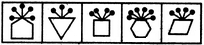(1)     (2)     (3)     (4)     (5)

Solution: The pins, equal in number to the number of sides in the main figure are attached to the midpoint of a side of the main figure in case of figures (2), (3), (4) and (5). In fig. (1), these pins are attached to a vertex of the main figure.
QUESTION: 2

### Directions to Solve In each problem, out of the five figures marked (1), (2), (3), (4) and (5), four are similar in a certain manner. However, one figure is not like the other four. Choose the figure which is different from the rest. Question - Choose the figure which is different from the rest.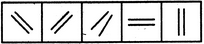(1)     (2)     (3)     (4)     (5)

Solution: In all other figures, the two line segments are parallel to each other.
QUESTION: 3

### Directions to Solve In each problem, out of the five figures marked (1), (2), (3), (4) and (5), four are similar in a certain manner. However, one figure is not like the other four. Choose the figure which is different from the rest. Question - Choose the figure which is different from the rest.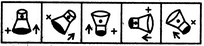(1)     (2)     (3)     (4)     (5)

Solution: In all other figures, the arrow and the V sign lie towards the black end of the main figure.
QUESTION: 4

In each problem, out of the five figures marked (1), (2), (3), (4) and (5), four are similar in a certain manner. However, one figure is not like the other four. Choose the figure which is different from the rest.

Question -

Choose the figure which is different from the rest.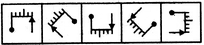(1)     (2)     (3)     (4)     (5)
Solution: In all other figures, there are two small line segments towards the pin and three small line segments towards the arrow.
QUESTION: 5

In each problem, out of the five figures marked (1), (2), (3), (4) and (5), four are similar in a certain manner. However, one figure is not like the other four. Choose the figure which is different from the rest.

Question -

Choose the figure which is different from the rest.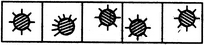(1)     (2)     (3)     (4)     (5)
Solution: The lines outside the shaded circle are not equally distributed along the circumference in fig. (2).
QUESTION: 6

In each problem, out of the five figures marked (1), (2), (3), (4) and (5), four are similar in a certain manner. However, one figure is not like the other four. Choose the figure which is different from the rest.

Question -

Choose the figure which is different from the rest.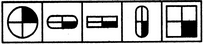(1)     (2)     (3)     (4)     (5)
Solution: In all other figures, the lower-right quarter portion is shaded.
QUESTION: 7

In each problem, out of the five figures marked (1), (2), (3), (4) and (5), four are similar in a certain manner. However, one figure is not like the other four. Choose the figure which is different from the rest.

Question -

Choose the figure which is different from the rest.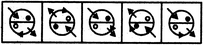(1)     (2)     (3)     (4)     (5)
Solution: Only in fig. (5), the arrowhead along the circumference of the circle indicates motion in an ACW direction.
QUESTION: 8

In each problem, out of the five figures marked (1), (2), (3), (4) and (5), four are similar in a certain manner. However, one figure is not like the other four. Choose the figure which is different from the rest.

Question -

Choose the figure which is different from the rest.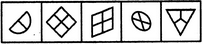(1)     (2)     (3)     (4)     (5)
Solution: All other figures are divided into equal parts.
QUESTION: 9

In each problem, out of the five figures marked (1), (2), (3), (4) and (5), four are similar in a certain manner. However, one figure is not like the other four. Choose the figure which is different from the rest.

Question -

Choose the figure which is different from the rest.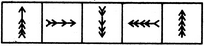(1)     (2)     (3)     (4)     (5)
Solution: Each one of the figures except fig. (2), consists of five arrowheads.
QUESTION: 10

In each problem, out of the five figures marked (1), (2), (3), (4) and (5), four are similar in a certain manner. However, one figure is not like the other four. Choose the figure which is different from the rest.

Question -

Choose the figure which is different from the rest.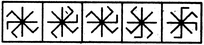(1)     (2)     (3)     (4)     (5)
Solution: Figure (1) and fig. (3) can be rotated into each other and fig. (4) and fig. (5) can be rotated into each other.
QUESTION: 11

In each problem, out of the five figures marked (1), (2), (3), (4) and (5), four are similar in a certain manner. However, one figure is not like the other four. Choose the figure which is different from the rest.

Question -

Choose the figure which is different from the rest.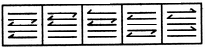(1)     (2)     (3)     (4)     (5)
Solution: In each one of the figures except fig. (5), two arrows point towards left and one arrow points towards right.
QUESTION: 12

In each problem, out of the five figures marked (1), (2), (3), (4) and (5), four are similar in a certain manner. However, one figure is not like the other four. Choose the figure which is different from the rest.

Question -

Choose the figure which is different from the rest.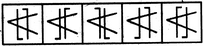(1)     (2)     (3)     (4)     (5)
Solution: Only in fig. (4), both the parallel lines are bent in the same direction {i.e. towards the left).
QUESTION: 13

In each problem, out of the five figures marked (1), (2), (3), (4) and (5), four are similar in a certain manner. However, one figure is not like the other four. Choose the figure which is different from the rest.

Question -

Choose the figure which is different from the rest.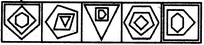(1)     (2)     (3)     (4)     (5)
Solution: In all other figures, as we move from the innermost to the outermost element, the numbers of sides of the elements either increase or decrease in a sequence.
QUESTION: 14

In each problem, out of the five figures marked (1), (2), (3), (4) and (5), four are similar in a certain manner. However, one figure is not like the other four. Choose the figure which is different from the rest.

Question -

Choose the figure which is different from the rest.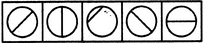(1)     (2)     (3)     (4)     (5)
Solution: Only in fig. (3), the line segment is not a diameter of the circle.
QUESTION: 15

In each problem, out of the five figures marked (1), (2), (3), (4) and (5), four are similar in a certain manner. However, one figure is not like the other four. Choose the figure which is different from the rest.

Question -

Choose the figure which is different from the rest.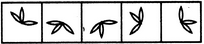(1)     (2)     (3)     (4)     (5)
Solution: All other figures can be rotated into each other.
QUESTION: 16

In each problem, out of the five figures marked (1), (2), (3), (4) and (5), four are similar in a certain manner. However, one figure is not like the other four. Choose the figure which is different from the rest.

Question -

Choose the figure which is different from the rest.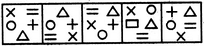(1)     (2)     (3)     (4)     (5)
Solution: Figure (4) has a rectangle in place of a '+' sign.
QUESTION: 17

In each problem, out of the five figures marked (1), (2), (3), (4) and (5), four are similar in a certain manner. However, one figure is not like the other four. Choose the figure which is different from the rest.

Question -

Choose the figure which is different from the rest.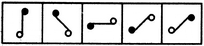(1)     (2)     (3)     (4)     (5)
Solution: All other figures can be rotated into each other.
QUESTION: 18

In each problem, out of the five figures marked (1), (2), (3), (4) and (5), four are similar in a certain manner. However, one figure is not like the other four. Choose the figure which is different from the rest.

Question -

Choose the figure which is different from the rest.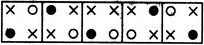(1)     (2)     (3)     (4)     (5)
Solution: In each one of the figures except fig. (3), the two crosses (x) appear in the diagonally opposite corners.
QUESTION: 19

In each problem, out of the five figures marked (1), (2), (3), (4) and (5), four are similar in a certain manner. However, one figure is not like the other four. Choose the figure which is different from the rest.

Question -

Choose the figure which is different from the rest.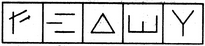(1)     (2)     (3)     (4)     (5)
Solution: Figure (4) is formed by four line segments while each one of the other figures is formed by three line segments.
QUESTION: 20

In each problem, out of the five figures marked (1), (2), (3), (4) and (5), four are similar in a certain manner. However, one figure is not like the other four. Choose the figure which is different from the rest.

Question -

Choose the figure which is different from the rest.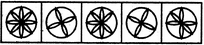(1)     (2)     (3)     (4)     (5)
Solution:
QUESTION: 21

In each problem, out of the five figures marked (1), (2), (3), (4) and (5), four are similar in a certain manner. However, one figure is not like the other four. Choose the figure which is different from the rest.

Question -

Choose the figure which is different from the rest.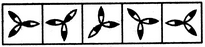(1)     (2)     (3)     (4)     (5)
Solution: Only in fig. (4), all the leaves have their outer halves shaded.
QUESTION: 22

In each problem, out of the five figures marked (1), (2), (3), (4) and (5), four are similar in a certain manner. However, one figure is not like the other four. Choose the figure which is different from the rest.

Question -

Choose the figure which is different from the rest.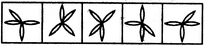(1)     (2)     (3)     (4)     (5)
Solution: All other figures can be rotated into each other.
QUESTION: 23

In each problem, out of the five figures marked (1), (2), (3), (4) and (5), four are similar in a certain manner. However, one figure is not like the other four. Choose the figure which is different from the rest.

Question -

Choose the figure which is different from the rest.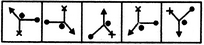(1)     (2)     (3)     (4)     (5)
Solution: In all other figures, the dot appears in the angle formed between the arrow and the pin.
QUESTION: 24

In each problem, out of the five figures marked (1), (2), (3), (4) and (5), four are similar in a certain manner. However, one figure is not like the other four. Choose the figure which is different from the rest.

Question -

Choose the figure which is different from the rest.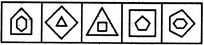(1)     (2)     (3)     (4)     (5)
Solution: In each one of the figures, except fig. (2), the number of sides in the inner element is one more than the number of sides in the outer element.
QUESTION: 25

In each problem, out of the five figures marked (1), (2), (3), (4) and (5), four are similar in a certain manner. However, one figure is not like the other four. Choose the figure which is different from the rest.

Question -

Choose the figure which is different from the rest.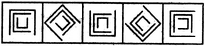(1)     (2)     (3)     (4)     (5)
Solution: All other figures can be rotated into each other. (In each figurer except fig, (1), the middle element is obtained by rotating the outer element through 90oCW and the inner element is obtained by rotating the middle element through 90oCW).